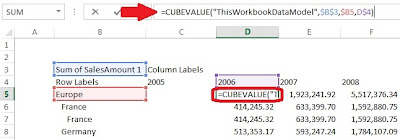Tuesday, August 6, 2013

P1.6: Notes on PivotTable Conversion to Formulas and Row-Column Oriented Databases

This post will touch on the nuances on what happens to a PivotTable is converted to formulas in Excel, and how PowerPivot uses column oriented databases. (Notes for P1.5).

When Converting to Formulas

When you convert a PivotTable to an Excel table using the Convert to Formulas button, Excel replaces the cells with formulas which reference the Data Model.Fig. 1: Convert to Formulas Button
For example when converted, the Internet sales table now has labels with from the formula CUBEMEMBER, and table values derived from CUBEVALUE.

CUBEMEMBER FunctionFig. 2: Sample Column Label Formula, CUBEMEMBER
Row and Column labels are retrieved from the Data Model or Cube by the CUBEMEMBER formula. The function accesses the the Data Model in this workbook which is generically called ThisWorkbookDataModel and to return the specific year of 2006, we need the table DimTime which has the FiscalYear. So the column formula for the year 2006 (Figure 2) is:
=CUBEMEMBER("ThisWorkbookDataModel","[DimTime].[FiscalYear].&")

CUBEVALUE FunctionFig. 3: Sample Table Value Formula, CUBEVALUE
The values in the table are retrieved through the Data Model, which is also a Cube. As with the CUBEMEMBER function, this particular CUBE function also accesses the the local Data Model in the workbook, ThisWorkbookDataModel. The specific value it finds is the value at \$B\$3, the sum of the SalesAmount. It further narrows it down to the territory group of Europe, which is in \$B5, while looking at year 2006, in cell D\$4. The CUBEVALUE function references a set of members as coordinates (hence, CUBEMEMBER for the row and column labels), which is how you would navigate OLAP Cubes. The table value formula for the sum of Internet sales in Europe in 2006 (Figure 3) is:
=CUBEVALUE("ThisWorkbookDataModel",\$B\$3,\$B5,D\$4)

MDX

Excel uses MDX to communicate with PowerPivot, and references the data in PowerPivot. The MDX syntax, such as CUBEVALUE, is similar to maneuvering in OLAP Cubes by providing a set of coordinates. In fact, PowerPivot stores the data as Cubes and are processed automatically.

Row and Column Oriented Databases

Row oriented databases, such as SQL Server, store data in a row fashion, which lists an entry with all the field values after. Generally row oriented databases have to scan all the rows to complete a query of an entire column of values, such as the summation of Sales. A complete table scan is required if you query one column or twenty columns, and with databases with many entries, it can be time consuming and not efficient.

On the other hand, column oriented databases, such as the xVelocity analytics engine in PowerPivot, stores data by column. If you query Sales in PowerPivot, it would only need to query the column of Sales. Data retrieval-wise, it is vastly more efficient to have a column oriented database because while querying a large entry dataset. You usually only analyze a few fields, mostly for an aggregate value, at a time. So querying a few fields (columns) would be more economical than querying all the rows for the few fields as in a row oriented database.

The most efficient query of a row oriented database would be a single entry row, since all the data for the row is stored together. It would be the most inefficient for a column oriented database because it would have to query all columns for that one row. In comparison, the most efficient query for a column oriented database would be a query of one column, which is also the most inefficient for the row oriented database because it would have to query all rows for a single field column.